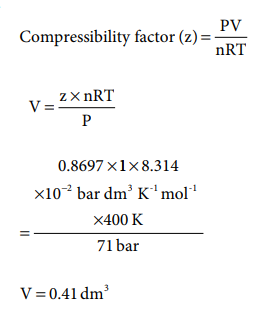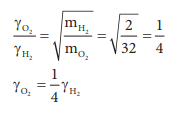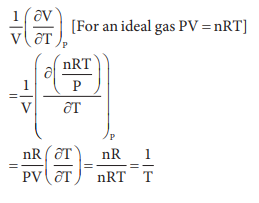Home | | Chemistry 11th std | Choose the best Answer: Gaseous State (Chemistry)

# Choose the best Answer: Gaseous State (Chemistry)

Chemistry : Gaseous State : Choose the best Answer

Gaseous State (Chemistry)

1. Gases deviate from ideal behavior at high pressure. Which of the following statement(s) is correct for non-ideality?

a) at high pressure the collision between the gas molecule become enormous

b) at high pressure the gas molecules move only in one direction

c) at high pressure, the volume of gas become insignificant

d) at high pressure the intermolecular interactions become significant

Answer: d) at high pressure the intermolecular interactions become significant

2. Rate of diffusion of a gas is

a) directly proportional to its density

b) directly proportional to its molecular weight

c) directly proportional to its square root of its molecular weight

d) inversely proportional to the square root of its molecular weight

Answer: d) inversely proportional to the square root of its molecular weight

3. Which of the following is the correct expression for the equation of state of van der Waals gas?4. When an ideal gas undergoes unrestrained expansion, no cooling occurs because the molecules

a) are above inversion temperature

b) exert no attractive forces on each other

c) do work equal to the loss in kinetic energy

d) collide without loss of energy

Answer: b) exert no attractive forces on each other

5. Equal weights of methane and oxygen are mixed in an empty container at 298 K. The fraction of total pressure exerted by oxygen is

(a) 1/3

(b) 1/2

(c) 2/3

(d) 1/3 ├Ś 273 ├Ś 298

Solution:

mass of methane

= mass of oxygen = a

number of moles of methane = a/16

number of moles of Oxygen = a/32

mole fraction of Oxygen =

=[a/32] / [{a/16} + {a/32{ ]

= [a/32] / [3a/32]

=1/32

Partial pressure of oxygen

= mole fraction Total Pressure

=1/3 P

6. The temperatures at which real gases obey the ideal gas laws over a wide range of pres-sure is called

a) Critical temperature

b) Boyle temperature

c) Inversion temperature

d) Reduced temperature

Solution:

The temperature at which real gases obey the ideal gas laws over a wide range of pressure is called Boyle temperature

7. In a closed room of 1000 m3 a perfume bottle is opened up. The room develops a smell. This is due to which property of gases?

a) Viscosity

b) Density

c) Diffusion

d) None

8. A bottle of ammonia and a bottle of HCl connected through a long tube are opened simultaneously at both ends. The white ammonium chloride ring first formed will be

a) At the center of the tube

b) Near the hydrogen chloride bottle

c) Near the ammonia bottle

d) Throughout the length of the tube

Answer: b) Near the hydrogen chloride bottle

Solution:

Rate of diffusion ╬▒ 1/ŌłÜm

mNH3 = 17 ; mHCl = 36.5

╬│NH3 > ╬│HCl

Hence white fumes first formed near hydrogen chloride

9. The value of universal gas constant depends upon

a) Temperature of the gas

b) Volume of the gas

c) Number of moles of the gas

d) units of Pressure and volume.

Answer: (d) pressure and volume of the gas

10. The value of the gas constant R is

a) 0.082 dm3atm.

b) 0.987 cal mol-1 K-1

c) 8.3 J mol-1K-1

d) 8 erg mol-1K-1

11. Use of hot air balloon in sports at meteorological observation is an application of

a) BoyleŌĆÖs law

b) NewtonŌĆÖs law

c) KelvinŌĆÖs law

d) BrownŌĆÖs law

12.  The table indicates the value of van der Waals constant ŌĆśaŌĆÖ in (dm3)2atm. mol-2The gas which can be most easily liquefied is

a) O2

b) N2

c) NH3

d) CH4

Solution:

Higher the value of 'a', greater the intermolecular force of attraction, easier the liquefaction.

13. Consider the following statements

i. Atmospheric pressure is less at the top of a mountain than at sea level

ii. Gases are much more compressible than solids or liquids

iii. When the atmospheric pressure increases the height of the mercury column rises Select the correct statement

a) I and II

b) II and III

c) I and III

d) I, II and III

Answer: d) I, II and III

14. Compressibility factor for CO2 at 400 K and 71.0 bar is 0.8697. The molar volume of CO2 under these conditions is

a) 22.04 dm3

b) 2.24 dm3

c) 0.41 dm3

d) 19.5dm3

Solution:15. If temperature and volume of an ideal gas is increased to twice its values, the initial pressure P becomes

a) 4P

b) 2P

c) P

d) 3P

Solution:16. At identical temperature and pressure, the rate of diffusion of hydrogen gas is 3ŌłÜ3 times that of a hydrocarbon having molecular formula CnH2nŌĆō2. What is the value of n ?

a) 8

b) 4

c) 3

d) 1

Solution:17. Equal moles of hydrogen and oxygen gases are placed in a container, with a pin-hole through which both can escape what fraction of oxygen escapes in the time required for one-half of the hydrogen to escape.

a) 3/8

b) 1/2

c) 1/8

d) 1/4

Solution:The fraction of oxygen that escapes in the time required for one half of the hydrogen to escape is 1/8

18. The variation of volume V, with temperature T, keeping pressure constant is called the coefficient of thermal expansion ie ╬▒ = 1/V (ŌłéV/ŌłéT)P. For an ideal gas ╬▒ is equal to

a) T

b) 1/T

c) P

d) none of these

Solution:19. Four gases P, Q, R and S have almost same values of 'b' but their 'a' values (a, b are Vander Waals Constants) are in the order Q < R < S < P. At a particular temperature, among the four gases the most easily liquefiable one is

a) P

b) Q

c) R

d) S

Solution:

Greater the 'a' value, easier the liquefaction

20. Maximum deviation from ideal gas is expected from

a) CH4 (g)

b) NH3(g)

c) H2 (g)

d) N2 (g)

21. The units of Vander Waals constants 'b' and 'a' respectively

a) mol LŌĆō1 and L atm2 molŌĆō1

b) mol L and L atm mol2

c) molŌĆō1L and L2 atm molŌĆō2

d) none of these

Answer: c) molŌĆō1L and L2 atm molŌĆō2

Solution:

an2/V2 = atm

= atm L2/mol2 = L2 mol-2 atm

nb = L

b= L/mol = L mol-1

22. Assertion : Critical temperature of CO2 is 304K, it can be liquefied above 304K.

Reason : For a given mass of gas, volume is to directly proportional to pressure at constant temperature

a) both assertion and reason are true and reason is the correct explanation of asser-tion

b) both assertion and reason are true but reason is not the correct explanation of assertion

c) assertion is true but reason is false

d) both assertion and reason are false

Answer: d) both assertion and reason are false

Solution:

Correct Statement: Critical temperature of CO2 is 304 K. It means that CO2 cannot be liquefied above 304 K, whatever the pressure may applied.

Pressure is inversely proportional to volume

23. What is the density of N2 gas at 227┬░C and 5.00 atm pressure? (R = 0.082 L atm KŌĆō1 molŌĆō1)

a) 1.40 g/L

b) 2.81 g/L

c) 3.41 g/L

d) 0.29 g/L

Solution:24. Which of the following diagrams correctly describes the behaviour of a fixed mass of an ideal gas ? (T is measured in K)Solution:

For a fixed mass of an ideal gas V ╬▒ T P ╬▒ 1/V

and PV = Constant

25. 25g of each of the following gases are taken at 27┬░C and 600 mm Hg pressure. Which of these will have the least volume ?

a) HBr

b) HCl

c) HF

d) HI

Solution:

At a given temperature and pressure

Volume ╬▒ no. of moles

Volume ╬▒ Mass / Molar mass

Volume ╬▒ 28 / Molar mass

i.e. if molar mass is more, volume is less. Hence HI has the least volume

Tags : with Answers and Solution , 11th Chemistry : UNIT 6 : Gaseous State
Study Material, Lecturing Notes, Assignment, Reference, Wiki description explanation, brief detail
11th Chemistry : UNIT 6 : Gaseous State : Choose the best Answer: Gaseous State (Chemistry) | with Answers and Solution